Next: Return code Up: Solving systems of distance Previous: Principle   Contents

Implementation

A system of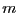distance functions has a specific description. First consider distance function that involve only points of constant. Such function may be written as: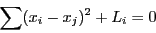where the maximal number of square term isand is described by a row of 2 matrices APOW, ACONS and a vector LI. APOW is an integer matrix with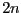columns androws that contain the unknown number of each term in the function and in which a 0 means that the unknown is a constant. The value of theses constants are given in the matrix of real ACONS of size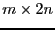. Finally the value of the constant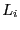is indicated in the vector LI of size. For example consider a system involving the 4 unknowns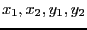numbered from 1 to 4: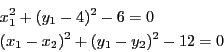In that case APOW, ACONS, LI will be: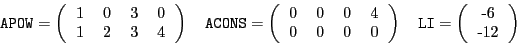The two first elements of the first row of APOW (1, 0) describes the first square term of the first equation and state that it is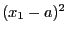, the value of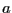being given in the ACONS(1,2). Note that each square term must be written as (unknown-unknown or constant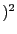and not as (constant -unknown.

Consider now function involving virtual points. Each square term may thus be written as: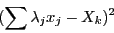where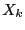may be either a constant, an unknown or the coordinate of a virtual point. Let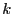be the number of term of the form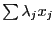existing in the system. Such equation is described by a matrix AVARV withrows and a number of columns equal to the number of unknowns. Each termis numbered from 1 to k and the row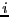of AVARV will contain in position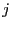the value of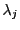. The existence of the coordinate of a virtual point in a distance function will be indicated in APOW by a negative number whose opposite is the number of the virtual coordinates. Hence if we add to the previous system the third equation: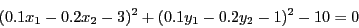the third row of APOW will be (-1 0 -2 0), the third row of ACONS will be (0 3 0 1) and the third row of LI will be -10. AVARV will have 2 row and 4 columns and is given as: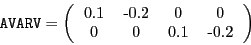Among theequations there will be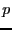equations involving virtual points. The system must be written in such way that first are defined the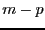equations not involving virtual points and then theequations. Note also that in the current implementation inequalities are handled although with less efficiency than equations. The algorithm is implemented as:

int Solve_Distance(int DimVar,int DimEq,
INTEGER_VECTOR &Type_Eq,
INTEGER_MATRIX &APOW,MATRIX &ACONS,VECTOR &LI,
int p,int k,MATRIX &AVARV,
INTERVAL_VECTOR & TheDomain,
int M,
double epsilonf,
int Stop,
INTERVAL_MATRIX & Solution,int Nb,
int (* Simp_Proc)(INTERVAL_VECTOR &))
The arguments are:
• DimVar: number of unknowns
• DimEq: number of equations and inequalities in the system
• Type_Eq: an integer array of dimension DimEq where the j-th element indicates if the j-th function is an equation (value =0), an inequality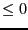(value = -1) or an inequality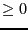(value = 1)
• p: number of functions involving virtual points
• k: number of virtual points
• TheDomain: box in which we are looking for solution of the system
• M: the maximum number of boxes which may be stored. In the algorithm we use the reverse storage mode except if the global integer ALIAS_Parallel_Slave is set to 1 (its default value is 0)
• epsilonf: either the maximal width of the function intervals for a solution if it is not determined by using Newton scheme or the accuracy used in the Newton scheme
• Stop: the possible values are 0,1,2
• 0: the algorithm will look for every solution in TheDomain
• 1: the algorithm will stop as soon as 1 solution has been found
• 2: the algorithm will stop as soon as Nb solutions have been found
• Solution: an interval matrix of size (Nb,m) which will contained the solution intervals. This list is sorted using the order specified by Order
• Nb: the maximal number of solution which will be returned by the algorithm
• Simp_Proc: a user-supplied procedure that take as input the current box and proceed to some further reduction of the width of the box or even determine that there is no solution for this box, in which case it should return -1 (see note 2.3.3). Remember also that you may use the 3B method to improve the efficiency of this algorithm (see section 2.3.2).
The argument Simp_Proc in this procedure may be omitted.

The following variables play also a role in the computation:

• ALIAS_Diam_Max_Kraw: if the maximal width of the ranges in a box is lower than this value, then the Krawczyk operator will be used to determine if there is a unique solution in the box (default value: 1.e10)
• ALIAS_Diam_Max_Newton: if the maximal width of the ranges in a box is lower than this value, then the interval Newton method will be used either to try to reduce the width of the box or to to ensure that there is no solution of the system in the box (default value: 1.e10)
• ALIAS_Permute_List: if the value of this flag isthe algorithm will permute the current list with the largest box in the list of boxes to process (as the algorithm uses systematically the Newton scheme with as initial guess the center of the current box permutation may allow to find quickly new solutions)

SubsectionsNext: Return code Up: Solving systems of distance Previous: Principle   Contents
Jean-Pierre Merlet 2012-12-20